NEET  >  31 Years NEET Previous Year Questions: Solutions - 1

# 31 Years NEET Previous Year Questions: Solutions - 1 - NEET

Test Description

## 17 Questions MCQ Test Chemistry Class 12 - 31 Years NEET Previous Year Questions: Solutions - 1

31 Years NEET Previous Year Questions: Solutions - 1 for NEET 2023 is part of Chemistry Class 12 preparation. The 31 Years NEET Previous Year Questions: Solutions - 1 questions and answers have been prepared according to the NEET exam syllabus.The 31 Years NEET Previous Year Questions: Solutions - 1 MCQs are made for NEET 2023 Exam. Find important definitions, questions, notes, meanings, examples, exercises, MCQs and online tests for 31 Years NEET Previous Year Questions: Solutions - 1 below.
Solutions of 31 Years NEET Previous Year Questions: Solutions - 1 questions in English are available as part of our Chemistry Class 12 for NEET & 31 Years NEET Previous Year Questions: Solutions - 1 solutions in Hindi for Chemistry Class 12 course. Download more important topics, notes, lectures and mock test series for NEET Exam by signing up for free. Attempt 31 Years NEET Previous Year Questions: Solutions - 1 | 17 questions in 35 minutes | Mock test for NEET preparation | Free important questions MCQ to study Chemistry Class 12 for NEET Exam | Download free PDF with solutions
 1 Crore+ students have signed up on EduRev. Have you?
31 Years NEET Previous Year Questions: Solutions - 1 - Question 1

### During osmosis, flow of water through a semi-permeable membrane  

Detailed Solution for 31 Years NEET Previous Year Questions: Solutions - 1 - Question 1

Osmosis is the phenomenon of flow of pure solvent from the solvent to the solution or from a less concentrated solution to a more concentrated solution through a semipermeable membrane. Common semipermeable membranes are permeable to certain solute particles also. In fact, there is no perfect semipermeable membrane. Therefore we can say that flow of water through a semipermeable membrane takes place on both sides with unequal rates.

31 Years NEET Previous Year Questions: Solutions - 1 - Question 2

### A solution containing 10 g per dm 3 of urea (molecular mass = 60 g mol–1) is isotonic with a 5% solution of a  non-volatile solute. The molecular mass of this nonvolatile solute is 

Detailed Solution for 31 Years NEET Previous Year Questions: Solutions - 1 - Question 2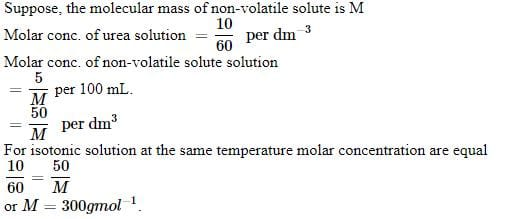31 Years NEET Previous Year Questions: Solutions - 1 - Question 3

### 1.00 g of a non-electrolyte solute (molar mass 250 g mol–1) was dissolved in 51.2 g of benzene. If the freezing point depression constant, Kf of benzene is 5.12 K kg mol–1, the freezing point of benzene will be lowered by 

Detailed Solution for 31 Years NEET Previous Year Questions: Solutions - 1 - Question 3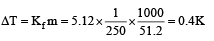31 Years NEET Previous Year Questions: Solutions - 1 - Question 4

0.5 molal aqueous solution of a weak acid (HX) is 20% ionised. If Kf for water is 1.86 K kg mol– 1,the lowering in freezing point of the solution is

Detailed Solution for 31 Years NEET Previous Year Questions: Solutions - 1 - Question 4

As ΔTf = iKfm

For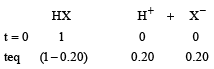Total no. of moles = 1 – 0.20 + 0.20 + 0.20 = 1 + 0.20 = 1.2
∴ ΔTf = 1.2 × 1.86 × 0.5 = 1.1160 ≈ 1.12 K

31 Years NEET Previous Year Questions: Solutions - 1 - Question 5

A 0. 00 20 m aqueous solution of an ionic compound Co(NH3)5(NO2)Cl freezes at – 0.00732 °C. Number of moles of ions which 1 mol of ionic compound produces on being dissolved in water will be (Kf = – 1.86°C/m)

Detailed Solution for 31 Years NEET Previous Year Questions: Solutions - 1 - Question 5

ΔTf = 0 – (0.00732°) = 0.00732
ΔTf = i × Kf × m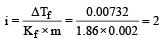31 Years NEET Previous Year Questions: Solutions - 1 - Question 6

An aqueous solution is 1.00 molal in KI. Which change will cause the vapour pressure of the solution to increase? 

Detailed Solution for 31 Years NEET Previous Year Questions: Solutions - 1 - Question 6

When the aqueous solution of one molal KI is diluted with water, concentration decreases, therefore the vapour pressure of the resulting solution increases.

31 Years NEET Previous Year Questions: Solutions - 1 - Question 7

A solution of sucrose (molar mass = 342 g mol–1) has been prepared by dissolving 68.5 g of sucrose in 1000 g of water. The freezing point of the solution obtained will be ( f for water = 1.86 K kg mol–1). 

Detailed Solution for 31 Years NEET Previous Year Questions: Solutions - 1 - Question 70.372
T = – 0.372°C

31 Years NEET Previous Year Questions: Solutions - 1 - Question 8

2 5. 3 g of sodium carbonate, Na2 CO3 is dissolved in enough water to make 250 mL of solution. If sodium carbonate dissociates completely, molar concentration of sodium ions, Na+ and carbonate ions, CO32– are respectively (Molar mass of Na2CO3 = 106 g mol–1) 

Detailed Solution for 31 Years NEET Previous Year Questions: Solutions - 1 - Question 8

Concentration of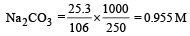[Na+] = 2 × 0.955 = 1.91 M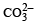= 0.955 M

31 Years NEET Previous Year Questions: Solutions - 1 - Question 9

The freezing point depression constant for water is – 1.86ºC m–1. If 5.00 g Na2SO4 is dissolved in 45.0 g H2O, the freezing point is changed by – 3.82ºC. Calculate the van’t Hoff factor for Na2SO4. 

Detailed Solution for 31 Years NEET Previous Year Questions: Solutions - 1 - Question 9

Given Kf = – 1.86º cm–1, mass of solute = 5.00 g, mass of solvent = 45.0 g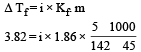∴ i = 2.63 (Molecular mass of Na2SO4 = 142)

31 Years NEET Previous Year Questions: Solutions - 1 - Question 10

The van’t Hoff factor i for a compound which undergoes dissociation in one solvent and association in other solvent is respectively : 

Detailed Solution for 31 Years NEET Previous Year Questions: Solutions - 1 - Question 10

If compound dissociates in solvent i > 1 and on association i < 1.

31 Years NEET Previous Year Questions: Solutions - 1 - Question 11

Mole fraction of the solute in a 1.00 molal aqueous solution is : 

Detailed Solution for 31 Years NEET Previous Year Questions: Solutions - 1 - Question 11

1 molal solution means 1 mole of solute dissolved in 1000 gm solvent.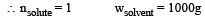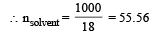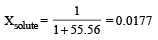31 Years NEET Previous Year Questions: Solutions - 1 - Question 12

A 0.1 molal aqueous solution of a weak acid is 30% ionized. If Kf for water is 1.86°C/m, the freezing point of the solution will be : [2011 M]

Detailed Solution for 31 Years NEET Previous Year Questions: Solutions - 1 - Question 12

Given α = 30% i.e., 0.3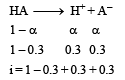i = 1.3
ΔTf = 1.3 × 1.86 × 0.1 = 0.2418
Tf = 0 – 0.2418 = – 0.2418 °C

31 Years NEET Previous Year Questions: Solutions - 1 - Question 13

200 mL of an aqueous solution of a protein contains its 1.26 g. The osmotic pressure of this solution at 300 K is found to be 2.57 × 10–3 bar.The molar mass of protein will be (R = 0.083 L bar mol–1 K–1)            [2011 M]

Detailed Solution for 31 Years NEET Previous Year Questions: Solutions - 1 - Question 13

(π) = CRT Osmotic pressure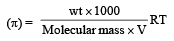= 2.57 10 3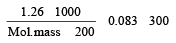Molecular mass = 61038 g

31 Years NEET Previous Year Questions: Solutions - 1 - Question 14

PA and PB are the vapour pressure of pure liquid components, A and B, respectively of an ideal binary solution. If XA represents the mole fraction of component A, the total pressure of the solution will be. 

Detailed Solution for 31 Years NEET Previous Year Questions: Solutions - 1 - Question 14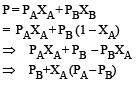31 Years NEET Previous Year Questions: Solutions - 1 - Question 15

Vapour pressure of chloroform (CHCl3) and dichloromethane (CH2Cl2) at 25ºC are 200 mm Hg and 415 mm Hg respectively. Vapour pressure of the solution obtained by mixing 25.5 g of CHCl3 and 40 g of CH2Cl2 at the same temperature will be : (Molecular mass of CHCl3 = 119.5 u and molecular mass of CH2Cl2 = 85 u).             [2012 M]

Detailed Solution for 31 Years NEET Previous Year Questions: Solutions - 1 - Question 15

The correct answer is option C
Molar mass of CH2​Cl2​ =12×1+1×2+35.5×2=85 g mol–1
Molar mass of CHCl3 ​  =12×1+1×1+35.5×3=119.5 g mol−1
Moles of CH2​Cl2​= 40 g /85 g mol−1 = 0.47 mol
Moles of CHCl3​ = 25.5 g /119.5 g mol−1 = 0.213 mol
Total number of moles = 0.47+0.213=0.683 mol
Mole fraction of component 2
=0.47mol/0.683mol= 0.688
Mole fraction of component 1
=1.00–0.688=0.312
We know that:
PT​=p10​+(p20​−p10​)x2​
=200+(415–200)×0.688
=200+147.9
=347.9 mm Hg

31 Years NEET Previous Year Questions: Solutions - 1 - Question 16

How many grams of concentrated nitric acid solution should be used to prepare 250 mL of 2.0M HNO3 ? The concentrated acid is 70% HNO3             [NEET 2013]

Detailed Solution for 31 Years NEET Previous Year Questions: Solutions - 1 - Question 16

Molarity (M)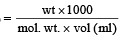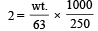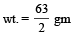wt. of 70% acid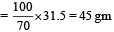31 Years NEET Previous Year Questions: Solutions - 1 - Question 17

Which condition is not satisfied by an ideal solution? [NEET Kar. 2013]

Detailed Solution for 31 Years NEET Previous Year Questions: Solutions - 1 - Question 17

An ideal solution is that solution in which each component obeys Raoult’s law under all conditions of temperatures and concentrations. For an ideal solution.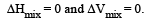## Chemistry Class 12

150 videos|373 docs|213 tests
Information about 31 Years NEET Previous Year Questions: Solutions - 1 Page
In this test you can find the Exam questions for 31 Years NEET Previous Year Questions: Solutions - 1 solved & explained in the simplest way possible. Besides giving Questions and answers for 31 Years NEET Previous Year Questions: Solutions - 1, EduRev gives you an ample number of Online tests for practice

## Chemistry Class 12

150 videos|373 docs|213 tests

### How to Prepare for NEET

Read our guide to prepare for NEET which is created by Toppers & the best Teachers